# Reverse words in a given String

## Introduction to the problem statement

You are given an N-length string. You need to reverse the string word by word. There can be multiple spaces between two words, as well as leading or trailing spaces. But the output should only be returning the reversed string with no extra spaces.

Let us take some examples to understand furthermore:

### Examples

Example 1: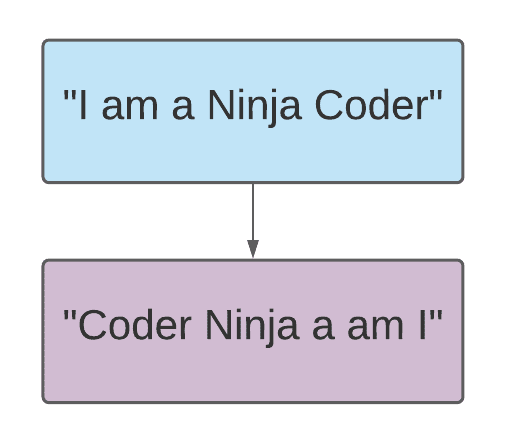Example 2: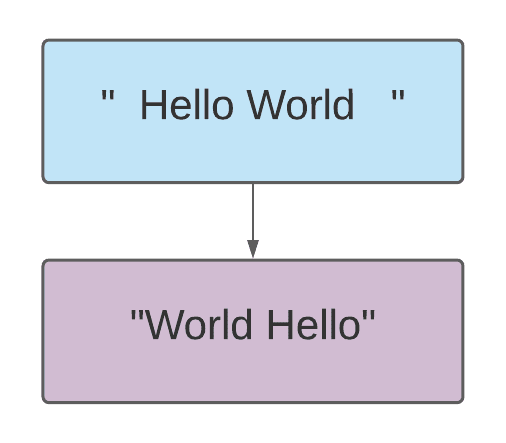Example 3: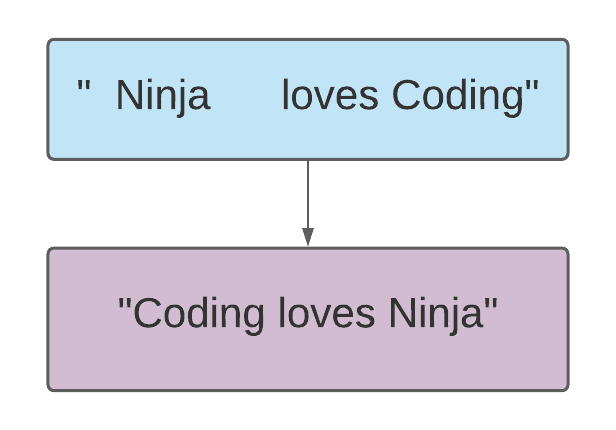Before you scroll further, it is recommended to try the given problem on your own.

Let us now discuss the methods to solve the above problem:

## Method 1: Brute force approach

The problem is to reverse words in a given string which means we need to extract words from the string and then reverse them. As we know, the words are separated by whitespaces. Using whitespaces, we’ll extract the words from the given string. The naive approach can be to maintain two pointers, the first one will only be incremented if there is whitespace and the other will only be incremented if there is a non-space character.

Let us understand with one example:The above diagram is an example string; now, our task is to extract the words by maintaining two pointers. Let us name them, i and j. Both will be initialized with 0.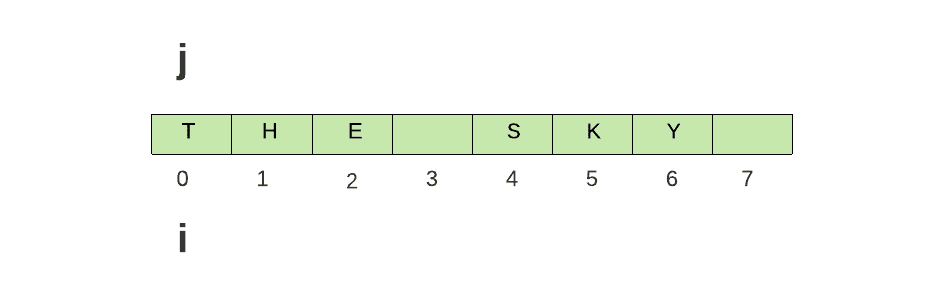Now, i will be incremented if it points to a space character, but there is no leading space; hence, it won’t be incremented.

Now, update the  j = i+1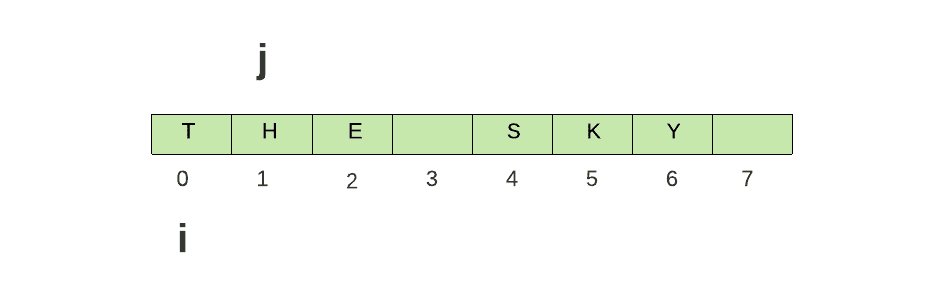The j pointer will be incremented if it points to the non-space character, as seen above. Now, increment the j until it encounters space.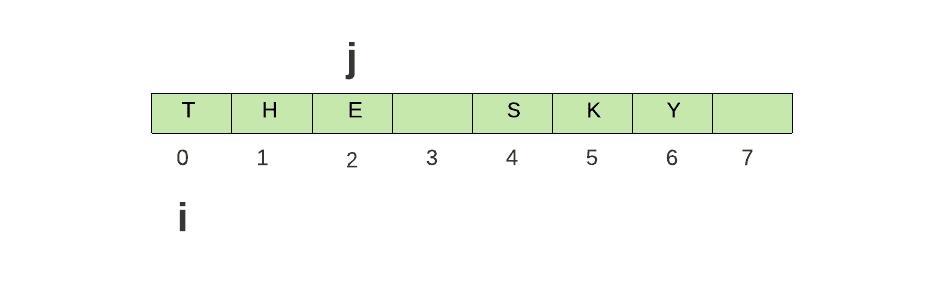Now, we have the starting and ending index of a word. Using the substring() method, pass starting and ending indexes and extract the word.

``String word = s.substring(i,j)``

To reverse words in a given string, create an empty string and store the extracted word like this:

``````String result = ""
result = word + " " + result``````

By doing so, each extracted word will be shifted at the end.

Lastly, to extract the rest of the words, update the i pointer.

``i = j+1``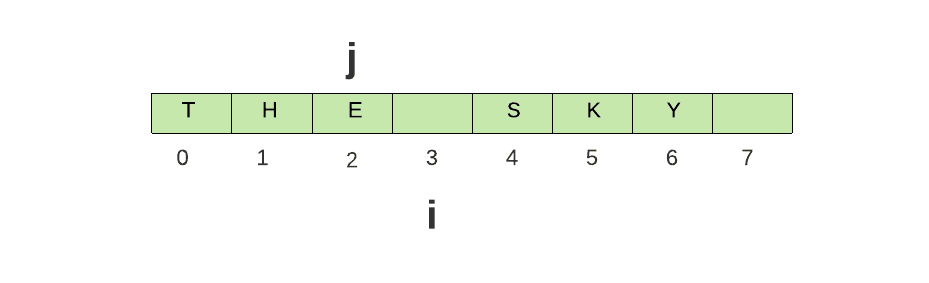Repeat the process until the end of the string is encountered.

After all the iterations, the result string will be as shown below:You can refer to the Pseudo Code given below to understand more:

Pseudo Code

``````public String reverseWords(String s)
Create an empty string
String res -> ""
int len -> s.length()
int i -> 0, j -> 0
while(i<len)
while(i<len and s.charAt(i)==' ')
i++
if(i==len)
break
j -> i+1;
while(j<len and s.charAt(j)!=' ')
j++
String word -> s.substring(i,j)
if(res.isEmpty())
res -> word + " "
else
res -> word + " " + res
i -> j+1;
return res``````

Let us now discuss the Implementation for the same:

### Implementation in java

``````// Brute force approach to reverse words in a given string

import java.io.*;
import java.util.*;

class CodingNinjas {
// function to reverse words in a given string
public static String reverseWords(String s) {
String res = "";
// take the given string's length
int len = s.length();
// Initialize two pointers to iterate over the string
int i = 0, j = 0;
// Iterate over the string until the end is reached
while (i < len) {
// Run two nested loops to get each word in a given string
// To do this, one pointer needs to care about the space character, and the second needs to care about the non-space character

// Here, i is taking care of the space character, increment i only when there is a space.
while (i < len && s.charAt(i) == ' ') {
i++;
}
// If i becomes equal to the length, break from the loop
if (i == len) {
break;
}
// i now must be pointing to a non-space character in a string, hence j is updated with i+1
// Here, j is taking care of the non-space character.
j = i + 1;
while (j < len && s.charAt(j) != ' ') {
j++;
}

// Now, i must be pointing to the starting of a word and j to the end of a word in a given string. To extract the word, use the substring() method and pass the starting and ending index into it.
String word = s.substring(i, j);

// Now, we need to reverse the string word by word-initially; we'll be getting the first word of a string, and to put it, at last, we add the new word first then the remaining words.
// Initially, the res string is empty. To avoid the extra space, we will add res = word + " "
if (res.isEmpty()) {
res = word + " ";
}
// If the res isn't empty, new words will be added at the beginning of the string.
else {
res = word + " " + res;
}

// Now, i must be updated as we need to encounter more words.
i = j + 1;
}
// finally return the reversed string
return res;
}
public static void main(String[] args) {
// String to be reversed
String sc = "I Love Coding Ninjas";
System.out.println("Original string : " + sc);
// Printing the reversed string.
System.out.println("Reversed string : " + reverseWords(sc));
}
}``````

Output

``````Original string : I Love Coding Ninjas
Reversed string : Ninjas Coding Love I ``````

### Complexity Analysis

Time Complexity: Since we are traversing each character in a string, the time complexity will be O(N), where N is the length of a given string.

Space Complexity: Since String is immutable in Java, we cannot modify or update the String once it is created. In the above Implementation, each time we tried to update the String, the formation of a new string occurred in the memory, which drastically poors the execution time.

To avoid the formation of new strings over and over again, Java offers an alternative to a String class, i.e., StringBuilder and StringBuffer class. Both the classes are mutable.

In this section, we’ll utilize the StringBuilder class to initialize the resultant String, and the rest will be the same as above.

## Method 2: Optimized brute force approach using String Builder class

Before we go into the solution, let us understand some key features of the StringBuilder class to grasp the concept in a better way:

• The StringBuilder class in java is used to create a mutable sequence of characters.
• There is no assurance of synchronisation with the StringBuilder class; hence it operates faster than the other classes.
• This class belongs to the java.lang package.
• It consumes less memory and is stored in the stack area.
• During the execution, its performance is very high.

Below is the example of an empty StringBuilder:

``StringBuilder sb = new StringBuilder();``

Let us now have a look at the Implementation part:

### Implementation in java

``````// Optimized approach by using StringBuilder class

import java.io.*;
import java.util.*;

class CodingNinjas {
// function to reverse words in a given string
public static String reverseWords(String s) {
// Declaration of a string using the StringBuilder class to make the execution.
StringBuilder res = new StringBuilder();

// take the given string's length
int len = s.length();
// Initialize two pointers to iterate over the string
int i = 0, j = 0;
// Iterate over the string until the end is reached
while (i < len) {
while (i < len && s.charAt(i) == ' ') {
i++;
}
if (i == len) {
break;
}
j = i + 1;
while (j < len && s.charAt(j) != ' ') {
j++;
}

String word = s.substring(i, j);
// Initially, as the res string is empty, to avoid the extra space append only the word, if this case is not handled then the output string would've extra spaces.
if (res.length() == 0) {
res.append(word);
} else {
// Insert the current word at the 0th location to reverse the string
// 0 is the offset and word +" " is the string
res.insert(0, word + " ");
}

i = j + 1;
}
// As the res is a type of String builder class, convert it as a string using the toString() method.
return res.toString();
}
public static void main(String[] args) {
// String to be reversed
String sc = "I Love Coding Ninjas";
System.out.println("Original string : " + sc);
// Printing the reversed string.
System.out.println("Reversed string : " + reverseWords(sc));
}
}``````

Output

``````Original string : I Love Coding Ninjas
Reversed string : Ninjas Coding Love I``````

### Complexity Analysis

Time Complexity: O(N), where N is the length of a given string.

Space Complexity: Since we are employing additional strings to return as a result, thus the space complexity will be O(N), where N is the length of a given string.

The other method could be using in-built functions. The below approach has no relation with the prior ones.

## Method 3: Using public String[] split( ) method

There is a special in-built method split() that can help us in solving the problem.

The string split() function splits a string into segments based on regular expression matching. This function produces a char array after breaking against the specified regular expression.

What is a regular expression?

A regular expression is a character-by-character search pattern. When searching for data in a text, this search pattern can be used to specify what you're looking for.

A regular expression might be as simple as a single character or as complex as a complex pattern.

For example:-

Input String: Coding@Ninjas

Regular Expression: @

Output: { "Coding", "Ninjas"}

Syntax of Split() method:

``split(String regex)``

It takes a regular expression as an argument and breaks the provided string around the regex matches.

Parameters: regex is a regular expression that serves as a delimiter.

Returns: Splitting the specified string produces an array of strings.

How can we use this to solve our problem if we notice we need to separate the words in a given string. We’ll use the split() method and pass whitespace as regex, but we have seen that there can be multiple spaces.

We will use the “\\ s +” operator to handle the multiple spaces, which indicates one or more spaces in a given string.

Let us look at an algorithm to do so:

The Algorithm says:

1. Create an empty StringBuilder, which we’ll return at the end of the function.
2. Using the in-built trim() function, trim the passed string by the main() function to have no leading or trailing spaces.
3. Apply the split() method to the trimmed string and pass “\\s+” as a regex delimiter.
4. Store the splitted array of strings in an additional array.
5. To reverse the words, use for-each or any other loop and append each array element into the StringBuilder s.
6. To return the string, use the toString() method and simply return the string to the main function.

Let us now have a look at the Implementation part:

### Implementation in java

``````import java.io.*;
import java.util.*;

public class CodingNinjas {
static String reverseWords(String sc) {
// If the string is empty return null
if (sc.isEmpty()) {
return null;
}
StringBuilder s = new StringBuilder();
// split() function splits the string into an array of strings.
// \\ s + - matches a sequence of one or more whitespace characters.
String arrOfStr[] = sc.trim().split("\\s+");
// To put the first word at last, yet again insert() would be helpful as it inserts the word at the first index and by maintaining the inserted strings.
for (String str: arrOfStr) {
if (s.length() == 0) {
s.append(str);
} else {
s.insert(0, str + " ");
}
}

// We could've done this to:- return s.toString().trim() to remove the extra spaces.
return s.toString();

}
public static void main(String args[]) {
String sc = " Hi Coders out     there    ";
System.out.println("Original String: \n" + sc);
System.out.println("Reversed String: \n" + reverseWords(sc));
}
}``````

Output

``````Original String:
Hi Coders out     there
Reversed String:
there out Coders Hi``````

### Complexity Analysis

Time Complexity: Let us break down each operation we’ve performed to understand the time taken for execution:

1. Trim(), split(), toString() , append(), insert() methods take o(N) time.
2. Traversal of each element in a string array will take o(N) time.

Total Time taken :- o(N) + o(N) + o(N) + o(N) + o(N) + o(N) ~ O(N).

Space Complexity: Since we are utilising an additional array and StringBuilder object, the space complexity will be O(N), where N is the length of a given string.

1. Why String is immutable in java?
A String is an immutable (cannot be modified once generated) object. The object created as a String is stored in the Constant String Pool. Since every immutable object in Java is thread-safe, String is likewise thread-safe. The String cannot be used by two threads at the same time.
2. What is the difference between String and StringBuilder in java?
Apart from mutability, When performing concatenations, StringBuilder is faster and uses less memory than a string. This is due to the fact that strings are immutable in Java, therefore concatenating two string objects requires the creation of a new object.
3. What is a regular expression in java?
A regular expression (regex) is a string search pattern. The search pattern might be as simple as a single letter, as complicated as a fixed string, or as a complex expression comprising special characters that describe the pattern.

## Key takeaways

Here we come at the end; nevertheless, the discussion is not over yet. Much more is being prepared specifically for you.

To warp up the session, we’ve explored three approaches to reverse words in a given string. Now, it is essential to dry run the approaches on your own only then you’ll get the concept. Understanding String-based problems are very needed as they are highly sought after in many companies.

Don’t stop here Nina, keep grinding the fantastic content we’ve prepared just for you.

Follow up the articles if you find any difficulty in solving any problem.

Thank you for reading; I hope you understood the concept well.

Have fun coding!